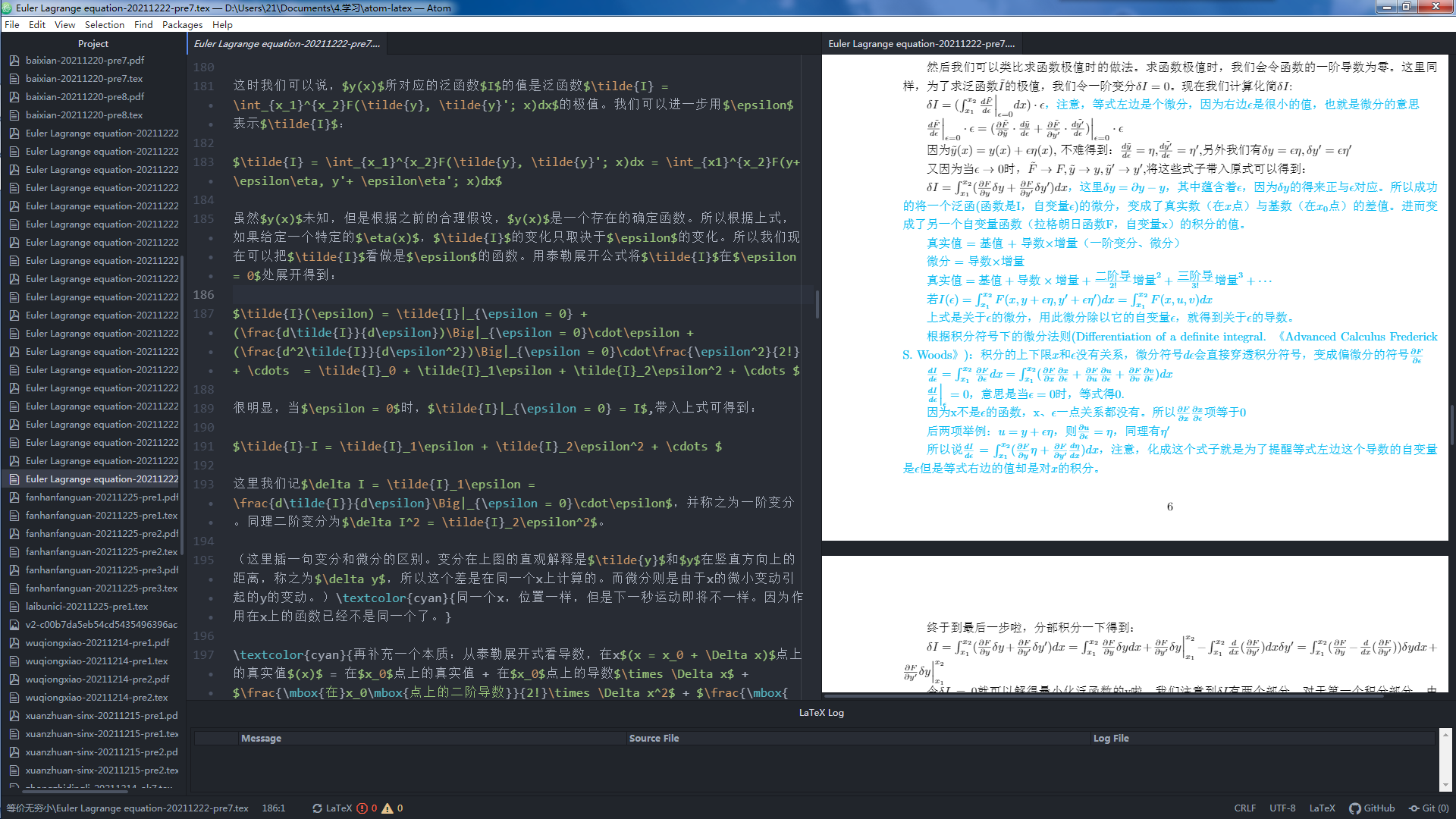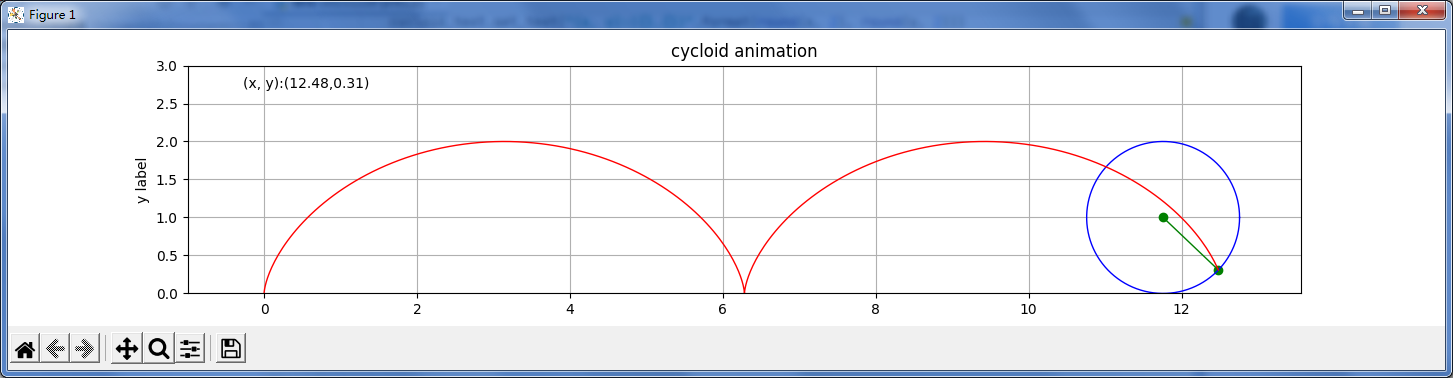# 导数4：摆线、最速降线的原理推导：欧拉-拉格朗日定理

995 2021-12-26 10:05

4-1.欧拉-拉格朗日方程

4-2.摆线、最速降线## 拉格朗日方程

d d t ∂ L ∂ q i ˙ − ∂ L ∂ q i = Q i {\displaystyle {\mathrm {d} \over \mathrm {d} t}{\partial {\mathcal {L}} \over \partial {\dot {q_{i}}}}-{\partial {\mathcal {L}} \over \partial q_{i}}=Q_{i}}·